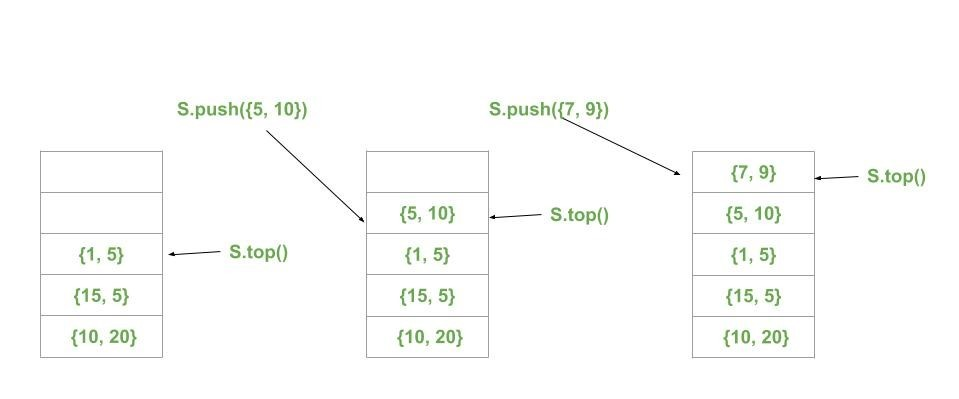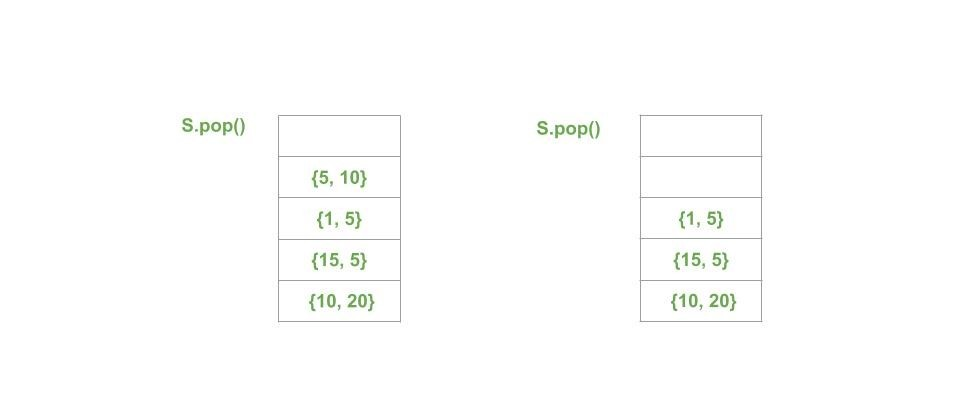Related Articles

# Stack of Pair in C++ STL with Examples

• Difficulty Level : Easy
• Last Updated : 25 Mar, 2020

Stack in STL Stacks are a type of container adaptors with LIFO(Last In First Out) type of working, where a new element is added at one end and (top) an element is removed from that end only.

Pair in STL The pair container is a simple container defined in header consisting of two data elements or objects. The first element is referenced as ‘first’ and the second element as ‘second’ and the order is fixed (first, second).

Stack of pair in STL: Stack of pair can be very efficient in designing complex data structures.

Syntax:

```stack<pair<datatype, datatype>> stack_of_pair;
```

Below is an example to show the Stack of Pairs:

 `// CPP program to demonstrate``// the working of STL stack of pairs`` ` `#include ``using` `namespace` `std;`` ` `// Print the current pair``void` `printPair(pair<``int``, ``int``> p)``{`` ` `    ``cout << ``"("``         ``<< p.first << ``", "``         ``<< p.second << ``") "``;``}`` ` `// Print the Stack of Pairs``void` `Showstack(stack > s)``{``    ``while` `(!s.empty()) {``        ``printPair(s.top());``        ``s.pop();``    ``}`` ` `    ``cout << ``'\n'``;``}`` ` `// Driver code``int` `main()``{``    ``stack > s;`` ` `    ``s.push({ 10, 20 });``    ``s.push({ 15, 5 });``    ``s.push({ 1, 5 });``    ``s.push({ 5, 10 });``    ``s.push({ 7, 9 });`` ` `    ``cout << ``"Stack of Pairs: "``;``    ``Showstack(s);`` ` `    ``cout << ``"\nSize of Stack of Pairs: "``         ``<< s.size();``    ``cout << ``"\nTop of Stack of Pairs: "``;``    ``printPair(s.top());`` ` `    ``cout << ``"\n\nRemoving the top pair\n"``;``    ``s.pop();`` ` `    ``cout << ``"Current Stack of Pairs: "``;``    ``Showstack(s);`` ` `    ``return` `0;``}`
Output:
```Stack of Pairs: (7, 9) (5, 10) (1, 5) (15, 5) (10, 20)

Size of Stack of Pairs: 5
Top of Stack of Pairs: (7, 9)

Removing the top pair
Current Stack of Pairs: (5, 10) (1, 5) (15, 5) (10, 20)
```

Below are the images to show the working of Stack of Pairs:Attention reader! Don’t stop learning now. Get hold of all the important DSA concepts with the DSA Self Paced Course at a student-friendly price and become industry ready.  To complete your preparation from learning a language to DS Algo and many more,  please refer Complete Interview Preparation Course.

In case you wish to attend live classes with experts, please refer DSA Live Classes for Working Professionals and Competitive Programming Live for Students.

My Personal Notes arrow_drop_up